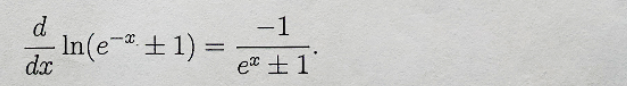## 2D ideal Fermi gas.

2D ideal Fermi gas
Consider an ideal Fermi gas in 2D. It is contained in an area of dimensions L x L. The particle mass is m

1. Find the density of states D(∈)
2. Find the Fermi energy as a function of the particle density n = N/L2
3. Find the total energy as a function of the Fermi energy ∈F
4. Find the chemical potential µ as a function of n and T

Hint:
Note thatSolved
General Physics 1 Answer Anonymous(s) Post